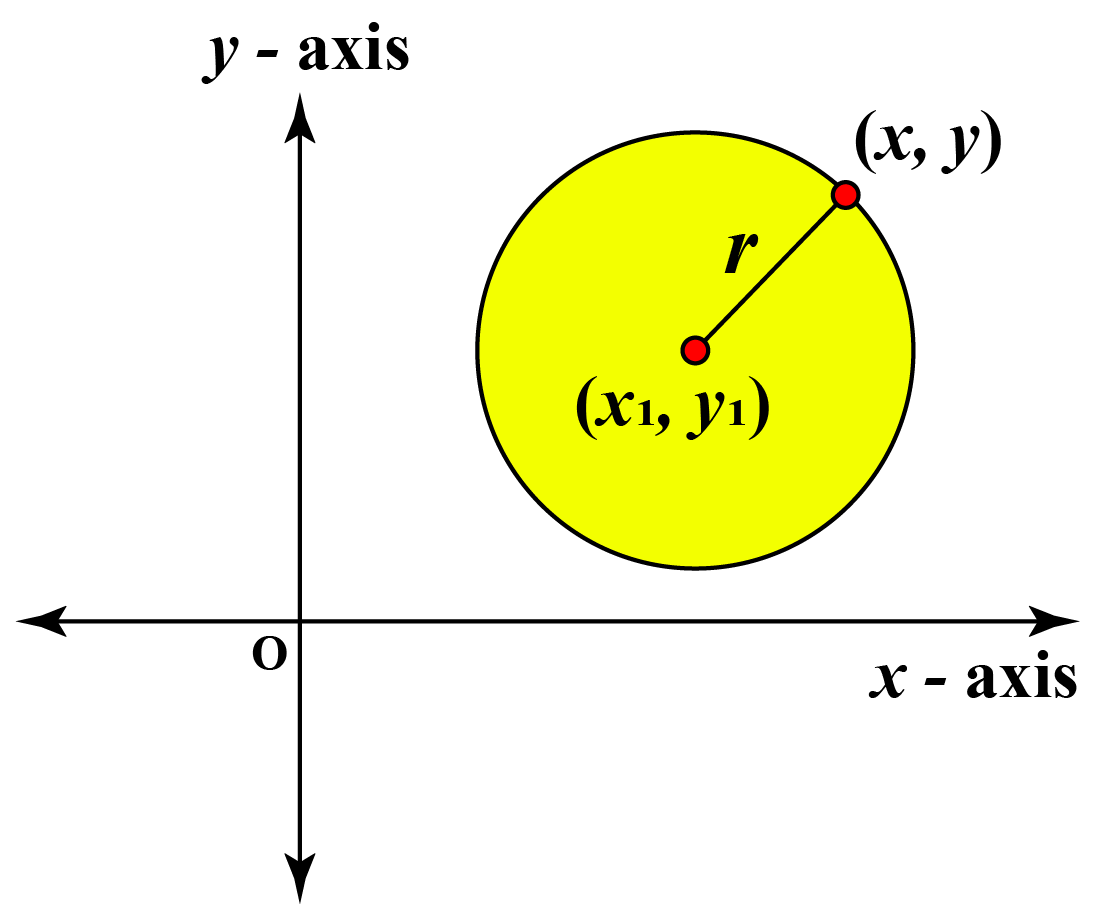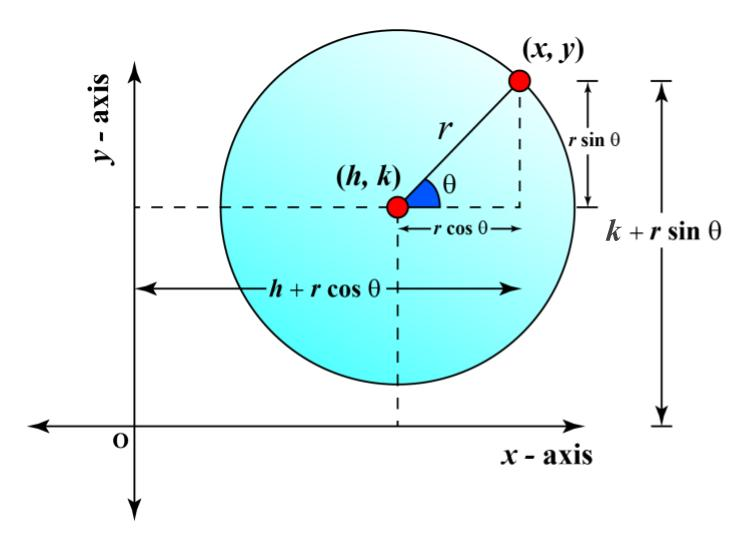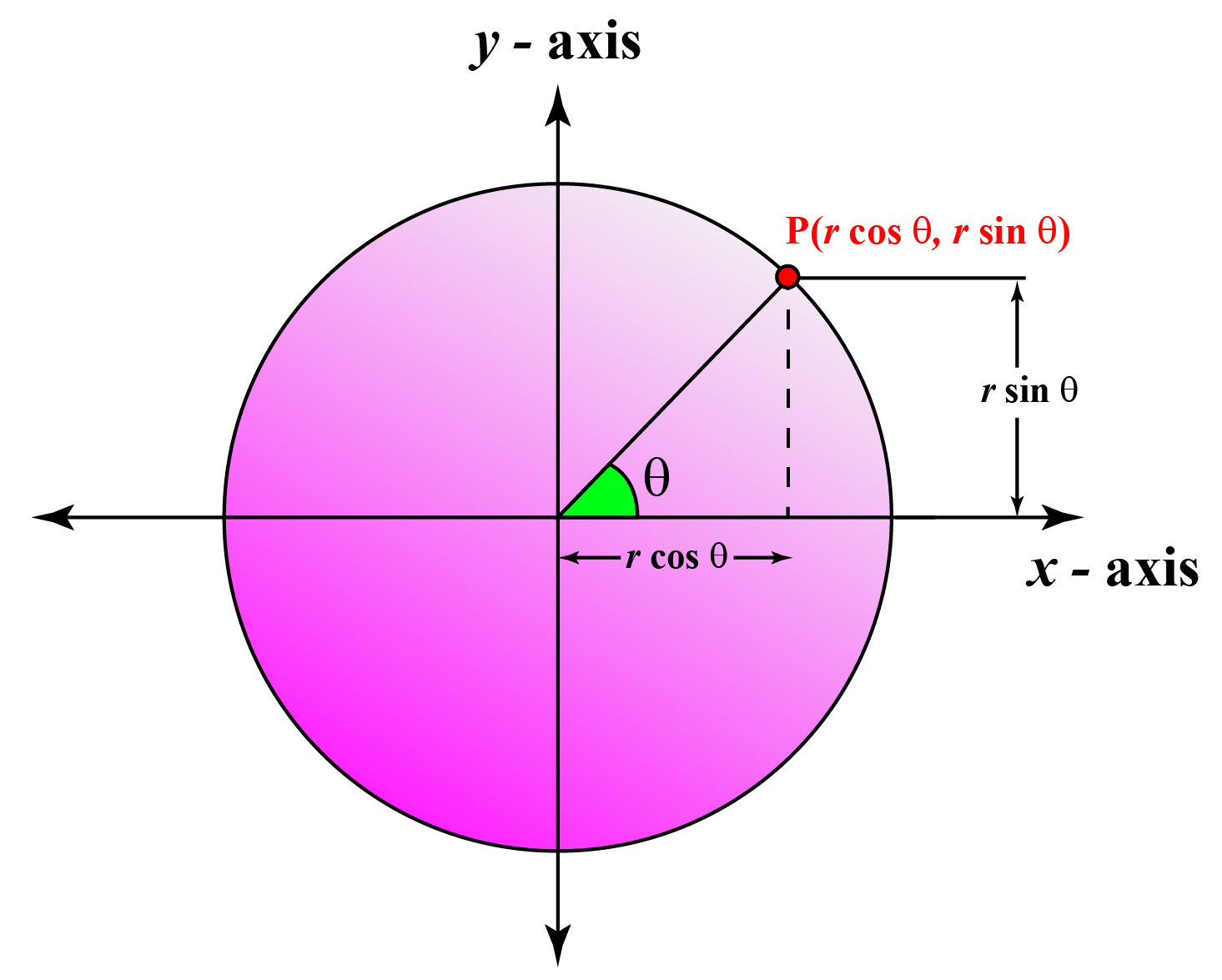# Equation of a Circle

In this mini-lesson, we will learn about the equations of a circle by understanding various forms of the equations of a circle, and how to apply them while solving problems. We will also discover interesting facts around them.

When we talk about the location of a point on the cartesian plane, we simply talk about the coordinates of that point.

For example, a point P having coordinate (1, 2) can easily be represented on the cartesian plane.

But what if we want to represent a circle on the cartesian plane?

To represent a circle on the cartesian plane, we require the equation of the circle.

A circle can be drawn on a piece of paper if we know its center and the length of its radius.

Similarly, on a cartesian plane, we can draw a circle if we know the coordinates of the center and its radius.

Explore the simulation below to get a basic idea of the equations of a circle.

Let's understand the equations of a circle in more detail.

## Lesson Plan

 1 What Are the Equations of a Circle? 2 Tips and Tricks 3 Important Notes on Equations of a Circle 4 Solved Example on Equations of a Circle 5 Interactive Questions on Equations of a Circle

## What Are the Equations of a Circle?

An equation of a circle represents the position of a circle in a cartesian plane.

If we know the coordinates of the center of the circle and the length of its radius, we can write the equation of a circle.

A circle can be represented in many forms:

• General form
• Standard form
• Parametric form
• Polar form

Let's explore each of these forms of the equation of a circle

## What Are the Various Forms of Equations of a Circle?

### General Form

The general form of the equation of a circle is:

 General form $x^2+y^2+Ax+By+C=0$

Where $$\text{A, B, C}$$ are constants.

If the center of the circle is $${(h, k)}$$ and the radius is $$r$$ then:

$$\text{A} = -2h$$
$$\text{B} = -2k$$
$$\text{C} = h^2 + k^2 - r^2$$

### Standard Form

Standard equation of a circle can be obtained if we consider $${(x_1, y_1)}$$ as the center of the circle with radius $$r$$.

$${(x, y)}$$ is an arbitrary point on the circumference of the circle.The distance between this point and the center is equal to the radius of the circle.

Let's apply the distance formula between these points.

$$\sqrt{(x - x_1)^2 + (y - y_1)^2} = r$$

Squaring both sides, we get:

$$(x - x_1)^2 + (y - y_1)^2 = r^2$$

This is the standard form of the equation of a circle.

 Standard form $(x - x_1)^2 + (y - y_1)^2 = r^2$

### Parametric Form

We know that the general form of equation of a circle is $$x^2 + y^2 + 2hx + 2ky + C = 0$$

We take a general point on the boundary of the circle, say $$(x, y)$$.

The line joining this general point and the center of the circle $$(h, k)$$ makes an angle of $$\theta$$.The parametric equation of circle can be written as $$x^2 + y^2 + 2hx + 2ky + C = 0$$ where $$x = -h +rcos \theta$$ and $$y = -k +rsin \theta$$

 Parametric form $x^2 + y^2 + 2hx + 2ky + C = 0$ $$x = -h +rcos \theta$$ and $$y = -k +rsin \theta$$

### Polar Form

The polar form of the equaion of circle is amost similar to the parametric form of the equation of circle.

We usually write the polar form of the equation of circle for the circle centered at the origin.

Let's take a point $$P(rcos{\theta}, rsin{\theta})$$ on the boundary of the circle, where $$r$$ is the distance of the point from the origin.We know that the equation of a circle centered at the origin and having radius $$p$$ is $$x^2 + y^2 = p^2$$

Substitute the value of $$x = rcos{\theta}$$ and $$y = rsin{\theta}$$ in the equation of circle.

${(rcos{\theta})}^2 + {(rsin{\theta})}^2 = p^2 \\ r^2 {cos}^2{\theta} + r^2 {sin}^2{\theta} = p^2 \\ r^2 ({cos}^2{\theta} + {sin}^2{\theta}) = p^2 \\ r^2 (1) = p^2 \\ r = p$

 Polar form $r = p$

Where $$p$$ is the radius of the circle.Tips and Tricks

• If a circle is represented in a polar form and its center is not at the origin, replace $$x$$ with $$rcos{\theta}$$ and $$y$$ with $$rsin{\theta}$$.
• To find the coordinates of the center of a circle in general form, just divide the negative of coefficients of $$x$$ and $$y$$ by 2.
The half of the negative of the coefficient of $$x$$ is the x coordinate of the center and the half of the negative of the coefficient of $$y$$ is the y coordinate of the center.
• If the coefficient of $$x$$ is zero it means that the center lies on the y-axis and if the coefficient of $$y$$ is zero it means that the center lies on the x-axis.

## Solved Examples on Equations of a Circle

 Example 1

Mathew wrote an equation of a circle in general form $$x^2 + y^2 + 6x + 8y + 9 = 0$$ and asked his friend to find the coordinates of the center and the radius of the circle. His friend is not able to solve the question. Help Mathew to find the solution.

Solution

Given the equation of the circle $$x^2 + y^2 +6x + 8y + 9 = 0$$

The general form of the equation of the circle with center $$(x_1, y_1)$$ and radius $$r$$ is $$x^2 + y^2 + Ax + By + C = 0$$
where $$A = -2x_1$$
$$B = -2y_1$$
$$C = {x_1}^2 + {y_1}^2 -r^2$$

From the equation of the circle $$x^2 + y^2 +6x + 8y + 9 = 0$$

\begin{align} A &= 6 \\[0.2cm] -2x_1 &= 6 \\[0.2cm] x_1 &= -3 \\[0.2cm] B &= 8 \\[0.2cm] -2y_1 &= 8 \\[0.2cm] y_1 &= -4 \\[0.2cm] C &= 9 \\[0.2cm] {x_1}^2 + {y_1}^2 -r^2 &= 9 \\[0.2cm] {-3}^2 + {-4}^2 -r^2 &= 9 \\[0.2cm] 9 + 16 -r^2 &= 9 \\[0.2cm] r^2 &= 16 \\[0.2cm] r &= 4 \\[0.2cm] \end{align}

 $$\therefore$$ Center of the circle is (-3, -4) and radius is 4 units.
 Example 2

Paul observed that the line $$y + x = 1$$ and the circle $${(x - 1)}^2 + {(y - 1)}^2 = 1$$ intersect at two points. Paul is not able to find the coordinates of these two points. Help him.

Solution

To find the coordinates of the points of intersection of the line and the circle, let's solve these two equations simultaneously.

Equation of line: $$y + x = 1$$

Equation of the circle: $${(x - 1)}^2 + {(y - 1)}^2 = 1$$

Put $$y = 1 -x$$ in the equation of circle
\begin{align} {(x - 1)}^2 + {(1 - x - 1)}^2 &= 1 \\[0.2cm] {(x - 1)}^2 + {(- x)}^2 &= 1 \\[0.2cm] {(x - 1)}^2 + x^2 &= 1 \\[0.2cm] {(x - 1)}^2 &= 1 - x^2 \\[0.2cm] {(x - 1)}^2 &= (1 - x)(1 + x) \\[0.2cm] {(x - 1)}^2 - (1 - x)(1 + x) &= 0\\[0.2cm] {(x - 1)}^2 + (x - 1)(1 + x) &= 0\\[0.2cm] (x - 1)(x - 1 + 1 + x) &= 0\\[0.2cm] (x - 1)(2x) &= 0\\[0.2cm] x - 1 &= 0\\[0.2cm] 2x &= 0\\[0.2cm] \end{align}
$x = 1 \text{ and } x = 0$
Substituting these value of x in the equation of line
$y + 1 = 1 \\ y = 0 \\ y + 0 = 1 \\ y = 1$
Hence, $$(1, 0), (0, 1)$$ are the two points of intersection of the line and the circle.

 $$\therefore$$ (1, 0), (0, 1)
 Example 3

Find the equation of the circle in the polar form provided that the equation of the circle in standard form is: $$x^2 + y^2 = 9$$.

Solution

To find the equation of the circle in polar form, substitute the values of $$x$$ and $$y$$ with:

$$x = rcos{\theta}$$
$$y = rsin{\theta}$$

\begin{align} x^2 + y^2 &= 9 \\[0.2cm] {(rcos{\theta})}^2 + {(rsin{\theta})}^2 &= 9 \\[0.2cm] r^2 {cos}^2{\theta} + r^2 {sin}^2{\theta} &= 9 \\[0.2cm] r^2 ({cos}^2{\theta} + {sin}^2{\theta}) &= 9 \\[0.2cm] r^2 (1) &= 9 \\[0.2cm] r &= 3 \end{align}

 $$\therefore$$ $$r = 3$$

Here are a few activities for you to practice.

## Let's Summarize

This mini-lesson targeted in the fascinating concept of the equations of a circle. The math journey around the equations of a circle starts with what a student already knows, and goes on to creatively crafting a fresh concept in the young minds. Done in a way that is not only relatable and easy to grasp, but will also stay with them forever. Here lies the magic with Cuemath.

At Cuemath, our team of math experts is dedicated to making learning fun for our favorite readers, the students!

Through an interactive and engaging learning-teaching-learning approach, the teachers explore all angles of a topic.

Be it worksheets, online classes, doubt sessions, or any other form of relation, it’s the logical thinking and smart learning approach that we at Cuemath believe in.

### 1. How do you graph a circle equation?

To graph a circle equation, first find out the coordinates of the center of the circle and the radius of the circle with the help of the equation of the circle.

Then plot the center on a cartesian plane and with the help of a compass measure the radius and draw the circle.

### 2. How do you find the general equation of a circle?

If we know the coordinates of the center of a circle and the radius then we can find the general equation of a circle.

For example, the center of the circle is (1, 1) and the radius is 2 units then the general equation of the circle can be obtained by substituting the values of center and radius.

The general equation of the circle is $$x^2 + y^2 + Ax + By + C = 0$$.

$$\text{A} = -2 \times 1 = -2$$
$$\text{B} = -2 \times 1 = -2$$
$$\text{C} = 1^2 + 1^2 - 2^2 = -2$$

Hence the general form of the equation of circle is $$x^2 + y^2 - 2x - 2y - 2 = 0$$.

### 3. How do you write the standard form of a circle?

The standard form of the equation of a circle is $$(x - x_1)^2 + (y - y_1)^2 = r^2$$

Where $$(x_1, y_1)$$ is the coordinate of the center of the circle and $$r$$ is the radius of the circle

### 4. How do you go from standard form to a general form of a circle?

Let's convert the equation of a circle: $${(x - 1)}^2 + {(y - 2)}^2 = 4$$ from standard form to gerenal form.

$${(x - 1)}^2 + {(y - 2)}^2 = 4 \\ x^2 + 1 - 2x + y^2 + 4 - 4y = 4 \\ x^2 + y^2 - 2x - 4y + 1 = 0$$

The above form of equation is the general form of the equation of circle.

### 5. What is C in the general equation of a circle?

The general equation of a circle is $$x^2 + y^2 + Ax + By + C = 0$$

The coordinates of the center is $$(\dfrac{-A}{2}, \dfrac{-B}{2})$$ and the radius is $$r$$ then  $$C$$ can be written as:

$$C = {(\dfrac{-A}{2})}^2 + {(\dfrac{-B}{2})}^2 - r^2$$

### 6. How do you write the standard form of a circle with endpoints?

Let's take the two endpoints of the diameter to be $$(1, 1), \text{ and } (3, 3)$$.

First, calculate the midpoint by using the section formula.

The coordinates of the center will be $$(2, 2)$$.

Secondly, calculate the radius by distance formula between $$(1, 1), \text{ and } (2, 2)$$

Radius is equal to $$\sqrt{2}$$.

Now, the equation of the circle in standard form is $${(x - 2)}^2 + {(y - 2)}^2 = 2$$.

### 7. What is the polar equation of a circle?

The polar equation of the circle with the center as the origin is $$r = p$$, where $$p$$ is the radius of the circle.

### 8. How do you find the center of a circle given two endpoints of the diameter?

The center can be obtained if the endpoints of a diameter are given.

For example, the two endpoints of the diameter are $$(-1, 0), \text{ and } (3, 4)$$

We can apply the section formula of $$1:1$$ ratio because the center divides the diameter into two equal parts.

The coordinates of the center will be $$(1, 2)$$.

### 9. How do you find the center of a circle on a graph?

The center of a circle can be found on the graph. First, draw two chords of the circle.

Then draw the perpendicular bisectors of these chords. The point of intersection of perpendicular bisectors is the required center of the circle.

### 10. How do you find the radius of a circle with the center?

To find the radius of the circle, we need two points. One is the center point and another is any arbitrary point on the boundary of the circle.

Radius cannot be found only with a center point.

Coordinate Geometry
Grade 9 | Questions Set 1
Coordinate Geometry
Grade 10 | Questions Set 1
Coordinate Geometry